# Thermoelectric Cooler Control Using the DS4830 Optical Microcontroller

### Abstract

This application note first briefly discusses the basic operation theory of a thermoelectric cooler (TEC) and its application in optical modules. Then it presents a digital approach to TEC control based on the DS4830 optical microcontroller. Mathematical analysis, algorithm implementation, firmware flowcharts, coding tips, and an example code are included to make this article a step-by-step guide for TEC control using the DS4830. Accuracy of ±0.1°C is readily achievable with TEC devices used in typical optical modules.

This application note is organized as follows. Part I introduces the basic operation theory of a thermoelectric cooler (TEC) and its application in optical modules. Part II presents the derivation of the digital filters that are used in digital TEC control, based on the DS4830 optical microcontroller. Algorithm implementation and coding tips are given in Part III. A digital filter coefficient calculator, lab results, and an example code are appended to the end of the document.

### Part I: Background Information

#### Principle of Operation of a TEC

A thermoelectric cooler (TEC) is a device based on the Peltier effect. It typically comprises two kinds of materials and transfers heat from one side of the device to the other while a DC current is forced through it. The side from which heat is removed becomes cold. Contrastingly, the side to which heat is moved becomes hot. When the current reverses its direction, the previously "cold" side becomes hot and the previously "hot" side becomes cold.

A TEC has no moving parts or working fluids, so it is very reliable and can be very small in size. TECs are used in many applications that require precision temperature control, including optical modules.

#### TEC's Application in Optical Modules

There are two main reasons why an optical module may need precision temperature control.

1. The laser needs to be cooled or heated to maintain its optical performance.
2. The laser needs to be set at a specific wavelength.

A well-controlled temperature is also required in dense wavelength division multiplexing (DWDM) for accurate channel spacing. Although multiple lasers are capable of driving a fiber at the same time to achieve large multichannel data rates, the laser wavelength needs to be tightly controlled to ensure correct channel spacing. Since the laser wavelength is temperature-dependent, the temperature must be well-controlled.

Because of the reasons above, temperature control is an important task for many optical applications. TECs are widely used in such applications thanks to their small sizes and ease of use.

### Part II: Mathematics Behind TEC Control

#### TEC Control Overview

Dual-Loop Control

A TEC is typically used as a heating or cooling element to control the temperature of a certain device, which could be a laser module. To achieve good performance, dual closed-loop (the thermal-loop and the current-loop) control is implemented in most applications. Figure 1 is a simplified system block diagram showing the basic idea of TEC control.The control loops in a typical laser application basically work as follows. First, a target temperature for the laser module is set according to application requirements. A temperature-sensing device, which is often a thermistor, senses the actual module temperature. The difference of the target temperature and the actual temperature is the temperature error. A thermal-loop controller takes this temperature error and goes through some control logic. The output of the thermal-loop controller is the target TEC current. Similar to the thermal loop, a current-sensing component senses the TEC current and compares it against the target TEC current. The difference is the current error. Next, the current error is supplied to the current-loop controller. The current-loop controller regulates the TEC drive circuitry to keep the actual TEC current close to the target value. By judiciously designing the controllers and the TEC drive circuitry, high-performance TEC control can be achieved.

#### Conventional Control Strategies and Implementation

Many TEC control strategies available on the market use analog devices, such as analog TEC controller/drivers and operational amplifiers (op amps), to realize the control logic. Although these circuits are well established, they have some drawbacks.

1. The analog implementation usually requires many components, which in turn requires more PCB area. Having more components also renders higher failure rates.
2. In analog approaches, the control thresholds and coefficients are set by discrete components. In order to achieve high control performance, components with tight tolerances need to be used, which increases cost. These components are subject to drift over time.
3. From a development point of view, it is not easy to modify a developed circuit to work for a new application. The component values are interdependent and one must change a number of components to make a modification.

#### Digital TEC Control Using the DS4830

The DS4830 is a 16-bit microcontroller with the necessary resources to make high-performance digital TEC control possible. The DS4830 features:

• 13-bit plus sign analog-to-digital converter (ADC) with 26-input mux
• 8-channel 12-bit digital-to-analog converter (DAC)
• 10 pulse-width modulation (PWM) channels with up to 12-bit resolution
• 32k words of flash memory and 1k words of SRAM
• Single cycle multiply-accumulate unit (MAC) with a 48-bit accumulator

Unlike analog TEC controllers, the DS4830 implements the control logic using digital signal processing (DSP) via firmware. This reduces the number of components needed and also makes the control parameters more accurate and repeatable. In addition, it is easier to modify the firmware to accommodate new applications than to change discrete components. Figure 2 is a system block diagram illustrating the digital TEC control approach.Below are some notes on the block diagram.

1. The temperatures are converted to and represented by voltages. Controlling the thermistor voltage is virtually controlling the laser module's temperature.
2. All the input signals are digitized before being processed. The set-point voltage and the thermistor voltage are converted using single-ended channels, while the TEC current and voltage are converted using differential channels for better performance.
3. Both the thermal-loop controller and the current-loop controller in Figure 2 are implemented digitally, which reduces the number of components used.
4. The thermal loop and current loop have different update periods. The thermal-loop update period is usually a multiple of the current-loop update period. This will be discussed in detail later.

In the next section, we will discuss the principle and operation of the thermal-loop controller.

### DS4830 TEC Control: Thermal Loop

The main function of the thermal-loop controller is to take the temperature error, i.e., the difference of the target temperature and the actual temperature of the laser module, and generate the target TEC current. This is illustrated in Figure 1. More detail on this is shown in Figure 2.

As pointed out earlier, temperatures are converted to and represented by voltages. In particular, the target temperature of the laser module is represented by the set-point voltage, which is denoted by vset. The thermistor is typically placed very close to the laser module so that the temperature of the thermistor is virtually the same as that of the laser module. Again, the thermistor temperature is represented by the voltage across the thermistor, vtherm. So controlling the temperature of the laser module is equivalent to controlling vtherm. Since the thermal-loop controller is digitally implemented, both the set-point voltage and the thermistor voltage are digitized by ADC.

#### An Analog Prototype for the Thermal-Loop Controller

A pragmatic way of developing a digital controller is to find the digital equivalent of a working analog controller. There are many analog control circuits available for TEC control applications, and Figure 3 is one of them. The circuit in Figure 3 takes vset and vtherm and generates vCTLI. The circuit is a proportional integral derivative (PID) controller. Its principle of operation is detailed in application note 3318, "HFAN-08.2.0: How to Control and Compensate a Thermoelectric Cooler (TEC)." The strategy here is first finding the continuous time transfer function of the circuit in Figure 3 and then converting the transfer function to the digital domain.#### Transfer Function of the Analog Controller in Figure 3

Based on the circuit shown in Figure 3, vCTLI can be expressed in terms of vset and vtherm. Since complex impedances are involved, it is convenient to introduce the complex variable s = j(2πf) = jω. Thus the impedance of a capacitor C1 is 1/(sC1). Taking advantage of basic op amp properties and some algebraic manipulations, we have the expression for vCTLI in s-domain as follows,

vCTLI(s) = GC(s)verr(s) + GF(s)vset(s)

where

verr(s) = vset(s) - vtherm(s)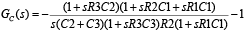Define

v1(s) = GC(s)verr(s)

v2(s) = GF(s)vset(s)

We can then rewrite vCTLI(s) as

vCTLI(s) = v1(s) + v2(s)

Now vCTLI(s) can be seen as the sum of the outputs of two single-input single-output (SISO) systems. Each system can be characterized by its transfer function, i.e., GF(s) and GF(s) respectively. The strategy here is to convert these two analog transfer functions to their digital equivalents, so they can be implemented by firmware. We use GC(z) and GF(z) to denote the digital equivalent of GC(s) and GF(s), respectively. This idea is illustrated in Figure 2.

There are several ways to convert analog systems to digital. Among them, bilinear transformation is a widely used one. The following section explains bilinear transformation in detail.

#### Bilinear Transformation

Bilinear transformation is entirely a frequency-domain method, and as a result, some of the optimal properties of the analog filter are preserved. In the following discussions, the time interval is denoted by the sampling interval T (in seconds). The bilinear transformation is a change of variables (a mapping) that is linear in both the numerator and denominator of a system transfer function . The usual form is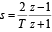The z-transform transfer function of the digital filters GC(z) and GF(z) are obtained from the corresponding Laplace transform transfer function GC(s) and GF(s) by substituting for s the bilinear form of Equation 8, respectively.

#### Obtaining Difference Equations for v1[n] and v2[n]

We obtain the analytical expressions for GC(z) and GF(z) by plugging Equation 8 into Equations 3 and 4, respectively. This is a straightforward process, but it requires numerous algebraic operations. Their final analytical expressions in terms of R/C values are given in Appendix A.

Finally, after inverse z-transform, digitized v1[n] and v2[n] are put into the conventional infinite impulse response (IIR) form shown below.Since this is a linear system, the superimposition property applies. We have

v_CTLI[n] = v1[n] + v2[n]Since this is a linear system, the superimposition property applies. We have

v_CTLI[n] = v1[n] + v2[n]

The coefficients in Equations 9 and 10 can be calculated using the Digital_Filter_Coeff_Cal spreadsheet (for more information, see Appendix B). Simply tweak the resistor and capacitor values at the top of the spreadsheet and the updated coefficients will be generated automatically at the bottom (in the yellow and purple cells).

#### Target TEC Current Calculation

Once we have v_CTLI[n], we can set the target TEC current using a simple linear mapping. If we use i_set[n] to denote the target TEC current, we haveRSENSE is the current sensing resistor shown in Figure 2.

The flow of the thermal-loop control can be summarized as follows. First, the target temperature is set according to system requirements. This temperature is represented by digital voltage v_set[n]. Then the thermistor furnishes the actual module temperature in the form of voltage, v_therm[n]. Next, the temperature error, v_err[n], is calculated by finding the difference of v_set[n] and v_therm[n]. v_CTLI[n] is then obtained by using Equations 9, 10, and 11. Finally, the target TEC current, i_set[n], is calculated using Equation 12 and furnished as the input to the current loop.

### DS4830 TEC Control: Current Loop

As we have described, TEC control comprises two loops: the thermal loop and the current loop. The thermal loop is the outer loop, and it generates the input to the current loop. The current loop is the inner loop, which is shown in Figure 2. The main function of the current loop is to regulate the TEC current to the target TEC current set by the thermal loop.

#### TEC Drive Circuitry

To facilitate description, we will review the TEC drive circuitry. Figure 4 is the functional block diagram for the TEC control and drive circuit.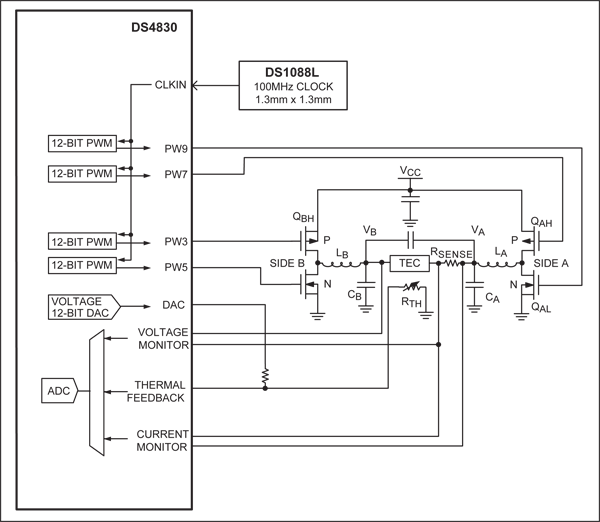In many applications, the TEC is required to provide both heating and cooling, but not at the same time, of course. This means that the drive circuit should be able to force current through the TEC in either direction. In addition, only a single supply is available in most applications. An H-bridge circuit can be used to drive a TEC with a single supply.

Figure 4 shows a simplified diagram for the H-bridge that is used to drive the TEC. The H-bridge comprises four MOSFETs, which are driven by four independent PWM signals generated by the DS4830. PW7 and PW9 drive MOSFETs QAH and QAL, respectively, and this side of the bridge is called "Side A." Similarly, PW3 and PW5 drive MOSFETs QBH and QBL on Side B, respectively. The MOSFETs on each side of the bridge, along with the corresponding inductor and capacitor, essentially form a synchronous buck converter. The two buck converters operate in-phase and in complementary mode to drive the TEC differentially. At zero TEC current, the differential voltage is zero, hence the buck converter outputs with respect to GND are equal to half of the supply voltage, which is 1.65V in our application. As the TEC current demand increases, one output will increase and the other will decrease from the initial point of 1.65V by an amount equal to half of the TEC voltage. Since TEC's volt-amp characteristic is largely resistive under normal operating conditions, we can regulate the TEC current by controlling the operating duty cycle of each buck converter.

Figure 2 shows how the current loop works. First, the target TEC current, i_set[n], is furnished by the thermal control loop. Then a current-sensing resistor samples the TEC current, i_TEC[n]. The difference of i_set[n] and i_TEC[n] is calculated and denoted as i_err[n]. Next, i_err[n] is furnished to a proportional integral (PI) controller. The output of the PI controller is e_PI[n] and it controls the buck converters' duty cycles. By controlling the buck converter's duty cycles, the current loop is able to regulate the TEC current to i_set[n].

#### PI Controller

A simplified current-loop flowchart is illustrated in Figure 5.The basic idea of a generic PI controller is as follows. If Vi(s) denotes the input of a PI controller and Vo(s) denotes the output, the transfer function of the controller can be written as:where KP is the proportional gain and KI is the integral gain. The values of KP and KI can be tweaked during debugging.

Using the bilinear transformation described earlier, Equation 13 can be converted to the digital domain and the corresponding difference equation has the following form:

vo[n] = -Acvo[n - 1] + Bc0vi[n] + Bc1vi[n - 1]

The coefficients can also be calculated using the Digital_Filter_Coeff_Cal spreadsheet. Note that the sampling period of the current loop is shorter than that of the thermal loop, typically by a factor of 8 or 10. In the example code, the value of the current-loop sampling period (Tc in the spreadsheet) is set to 1ms as opposed to 10ms sampling period for the thermal loop.

In our application, the input to the PI controller is i_err[n] and the output is e_PI[n]. We can then rewrite Equation 14 as

e_PI[n] = -Ace_PI[n - 1] + Bc0i_err[n] + Bc1i_err[n - 1]

Once we obtain e_PI[n], we can determine the PWM duty cycles accordingly. The following section explains the procedures.

#### Determine PWM Duty Cycles

We take a closer look at the H-bridge shown in Figure 4. The output voltage of the buck converter on Side A, VA, can be approximately expressed as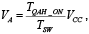where TSW is MOSFET QAH's switching period and TQAH_ON is the "on" time for QAH. Here we neglect the "on" drop of QAH and QAL. From Equation 16 we can see that at a certain switching frequency, the longer "on" time for QAH, the higher VA. Similarly, the output voltage of the buck converter on Side B, VB, can be approximately expressed aswhere TQBH_ON is the "on" time for QBH.

The voltage across the TEC is VA - VB - VRSENSE, where VRSENSE is the voltage across the current-sensing resistor, RSENSE. Therefore, by manipulating the "on" times of QAH and QBH, we can control the TEC voltage and thus the TEC current.

As shown in Figure 4, there are four MOSFETs and each of them is driven by a PWM signal from the DS4830. Table 1 summarizes the PWM signals and the MOSFETs they drive.

 MOSFETs Driven PWM Drive Signals MOSFET On-Time/Period Designator Topological Location Type Signal Name Duty Cycle QAH Side A high P channel PW7 D_AH 1 - D_AH QAL Side A low N channel PW9 D_AL D_AL QBH Side B high P channel PW3 D_BH 1 - D_BH QBL Side B low N channel PW5 D_BL D_BL

Note we define duty cycle as the high time over the period. For n-channel MOSFETs QAL and QBL, their on-time is equal to the high time of the PWM drive signals. Conversely, for p-channel MOSFETs, their on-time is equal to the low time of the PWM signals. These relationships are summarized in the MOSFET On-Time/Period column in Table 1.

Figure 6 shows the phases and duty cycles of the four PWM signals. Below are a few notes.

1. All PWMs have the same frequency.
2. For a certain side, the high-side MOSFET and the low-side MOSFET should never be "on" at the same time.
3. To further prevent shoot-through, a short dead-time is inserted between the high-side "on" stage and the low-side "on" stage.
4. Both Side A and Side B are synchronous buck converters. Together they drive the TEC differentially; thus their duty cycles are complementary. According to Figure 6, all other three duty cycles can be expressed in terms of D_AH. If we let DT denote the ratio between the dead time and the PWM period, we have:

D_AL = D_AH - 2 × DT

D_BH = 1 - D_AH

D_BL = D_BH - 2 × DT = 1 - D_AH - 2 × DT

Based on Equations 18 to 20, once we determine D_AH, all other three duty cycles can be calculated using simple math.Before we explain how to determine D_AH, we define the TEC current flowing from Side A to Side B as positive. Similarly, we define the TEC voltage as positive when VA > VB. With these two directions defined, we can move on and explain the strategy to determine D_AH.

Assume for a moment that the TEC current i_TEC[n] is less than the target TEC current i_set[n]. Then the current error i_err[n] = i_set[n] - i_TEC[n] is greater than zero. Subject to the proportional and integral gains, this positive i_err[n] will gradually drive the output of the PI controller e_PI[n] up.

Consequently, as shown in Figure 4, VA needs to increase and VB needs to decrease in order for i_TEC[n] to catch up with i_set[n]. Reviewing Equation 16 and Equation 17, we can see that the "on" time of QAH needs to increase in order for VA to go up. Also, the "on" time of QBH needs to decrease in order for VB to come down. This is how the buck converters on Side A and Side B work differentially. As shown in Figure 6, QAH's and QBH's "on" times are complementary and thus one can be expressed in terms of the other. In the following discussions, we will focus on the control of QAH's "on" time, TQAH_ON, as the "on" times or duty cycles of other three MOSFETs can be obtained in terms of TQAH_ON as illustrated in Table 1 and Equations 18 to 20.

To summarize the analysis above, a less than target TEC current results in a positive i_err[n], which drives e_PI[n] up, and a longer TQAH_ON is needed to boost the TEC current. Figure 7 illustrates the intuitive relationship between e_PI[n] and TQAH_ON for a closed-loop TEC current control.The fundamental trend is the larger e_PI[n], the longer TQAH_ON. Note that we have introduced limits (e_max and e_min) for e_PI[n] and TQAH_ON to avoid integral windup and reduce overshooting. e_PI[n] will be clamped to e_max if it becomes greater than e_max. Similarly, if e_PI[n] goes below e_min, it will be clamped to e_min.

Although the duty cycle of the two buck converters can run from 0% to 100%, it is good practice to keep the PWM duty cycles within a certain range. As shown in Figure 7, T_min denotes the minimum available "on" time for QAH and it corresponds to e_min. At the other end, T_max denotes the maximum available "on" time for QAH and it corresponds to e_max.

We assume TQAH_ON increases linearly as e_PI[n] increases. Then it is simple math to calculate TQAH_ON according to Figure 7.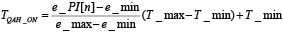Once TQAH_ON is determined using Equation 21, D_AH can be calculated using the relationship shown in Table 1. Then all other three PWM duty cycles can be obtained using Equations 18 to 20. Then one can set up the PWM channels and drive the TEC accordingly.

The following section of the document explains how to implement the control algorithms outlined above and provides some tips on coding.

### Part III: Algorithm Implementation and Coding Tips

So far we have elaborated the mathematical principles in dual-loop H-bridge TEC control. Next, we will discuss the firmware implementation and coding tips based on the DS4830.

### Top-Level Flowchart

Figure 8 is a top-level flowchart for the dual-loop H-bridge TEC control. Most of the blocks are straightforward and self-explanatory. There is one aspect, however, that needs elaboration: time allocation for the thermal loop and the current loop.

As we have discussed in Part II, TEC control using the DS4830 is essentially based on digital filtering. The two loops are updated periodically based on their respective set-points and feedback signals. Since the thermal loop is the outer loop and has longer response time, it has a longer update period. The current loop, comparatively, is the inner loop and needs a shorter update period. In one of our experiments, we empirically set the thermal-loop update period as 10ms and the current-loop update period as 1ms. This setting works well.

An important rule of digital filter updating is that the update period should be kept consistent. This is achieved by using a programmable timer. In the example code, we set up the Timer 1 in the DS4830 in such a way that it interrupts every 1ms, which is the current-loop-update period. Whenever Timer 1 interrupts, a new round of current-loop updates starts. A current-loop counter (c_lp_counter) ensures that the thermal-loop updates once every 10 times the current loop updates.

Figure 9 shows the processing time allocated to the dual loop updating. Figure 9(a) basically shows the time line for thermal-loop updating. For each round of thermal-loop updates, 10ms is available. By the end of each thermal-loop period, an updated target TEC current must be generated and output to the current-loop. Assume the current-loop updating starts at 0ms. A full round of thermal-loop updates must take place before 0ms so that there is an updated v_CTLI available for current-loop updating. Note that we drop the index ([n]) of v_CTLI without confusion (as opposed to v_CTLI[n] in Part II) because the index is not needed in coding.

As shown in Figure 9(b), the current loop is updated 10 times as fast as the thermal loop in our setup. In order for the DS4830 to update the two loops on time, we divide each current-loop-update period into 10 "coding slots." Each coding slot has 100µs, which is illustrated in Figure 9(c). The usage of each coding slot needs to be judiciously determined.

The first five coding slots in each current loop are dedicated for current-loop updating. In this way, the actual update period is tightly controlled as 1ms. It has been proven in debugging that 500µs is sufficient for one full round of current-loop updating. Therefore, each 1ms current-loop update period has 500µs available for other processing tasks. We use some of this time for thermal-loop updating.

We chop the thermal-loop-update code into four parts and allocate them to the last four coding slots in a current-loop-update period. This is illustrated in Figure 8 and Figure 9(c). It has been confirmed in debugging that the thermal-loop update receives sufficient processing time using this approach. This method ensures that the thermal loop is updated once in 10ms while the current loop is updated every 1ms.

The TEC control example code was coded in C. We will discuss the functions and operations used in the example code in the following sections.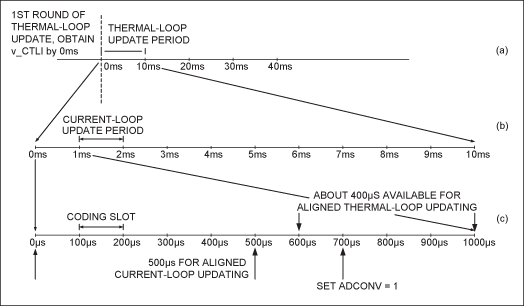### Programmable Timer and Associated Interrupt Service Routine (ISR)

As described briefly in the previous section, the firmware uses Timer 1 to generate periodic interrupts. We use a programmable timer function to fulfill this task. This is a simple function and the flowchart is shown in Figure 10.

Below is the description for the programmable timer function.

Function: void ProgTimer1(unsigned char k_c)

Input: unsigned char k_c. k_c can be any positive integer between 1 and 255.

Output: none

Description: This function sets up Timer 1 to interrupt every k_c × 100µs.

In our setup, we set k_c to 10 so Timer 1 interrupts every 1ms. The configurations of Timer 1 are clearly commented in the example code and Figure 10 illustrates the flowchart. We can use the following equation to find the correct reload value, which is denoted by X here.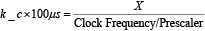Once Timer 1 interrupts, the ISR will be executed. The ISR will do the following:

1. Set the current-loop timer flag (CTIMER = 1) to mark the beginning of a new current-loop period.
2. Increment the current-loop counter (c_lp_counter) by 1. If the c_lp_counter is greater than 9, then reset it to 0. This marks the beginning of a new thermal-loop period. If the c_lp_counter is less than or equal to 9, then it means that the code has not finished the ongoing thermal loop.
3. Clear Timer 1's interrupt flag.
4. It is good practice to toggle a GPIO pin (P0.5 in the example code) every time Timer 1 interrupts. In this way, we can monitor the interrupt period by probing that GPIO pin using an oscilloscope. This step, however, has no effect on TEC control and is purely optional.

With Timer 1 set up and running, the updates can then be aligned correctly.### Using the Analog-to-Digital Converter (ADC)

There are four signals that need to be converted to digital: the TEC current, the TEC voltage, the set-point voltage (corresponding to the target temperature), and the thermistor voltage (corresponding to the actual temperature). Using the ADC to convert these signals to digital comprises two aspects: (1) setting up the ADC to perform the desired conversions, and (2) reading ADC data after the conversion is complete. We program different functions for ADC setup and data reading.

In the firmware, we use function SetupADC_DLP() to set up the ADC. Below is the description and Figure 11 shows the flowchart. We will discuss how to read ADC data in the next section.

Input: none.

Output: none.

Description: Overall, the ADC is set up to run in single-sequence mode. This means that the ADC must be re-enabled before a new sequence can be converted. Each channel will be converted multiple times and the average will be used for digital filtering. In each sequence, channel ADC_D0 is first converted eight times, followed by four conversions on ADC_D3, four conversions on ADC_S14, and finally four conversions on ADC_S15. In order to convert as fast as possible, the ADC clock is set to 1/8 of the core clock. All channels have acquisition extension disabled, and all ADC data are right aligned. Alternate result locations are used for auto-incremented sequential data reading. No data available interrupts will be generated.

To configure the ADC for each conversion, the code first sets the ADIDX register that is the index for each conversion. Then the configuration is written to the ADDATA register. Table 2 lists the desired configuration for each ADC channel.

Based on the discussion in Part II, the ADC must furnish a new set of TEC current and voltage data at the beginning of each current-loop-update period. This is necessary for accurate and stable digital control. In other words, it is important to align the new TEC current and voltage data with the update intervals. The shortest time per ADC conversion is 24.8µs. Considering that there are 12 conversions in total for the TEC current and voltage signals, we calculate that the total conversion time for ADC_D0 and ADC_D3 is approximately 300µs. Since ADC_D0 and ADC_D3 are the very first two channels to be converted, the TEC current and voltage data will become available at about 300µs after the ADC starts. So we start the ADC (by setting ADCONV = 1) 700µs after Timer 1 interrupts every time. In this way the TEC current and voltage will become available at the beginning of each following current-loop-update period, as shown in Figure 9(c).Out of the four signals being converted by the ADC, an updated TEC current and an updated TEC voltage are needed for every round of current-loop update (i.e., every 1ms). In contrast, the set-point voltage and the thermistor voltage are needed for every round of thermal-loop update (i.e., every 10ms). In light of the different update periods, we program two ADC-data-reading functions: ReadADC_IV() and ReadADC_ST().

ReadADC_IV() is called at the beginning of every round of current-loop update. This function only reads the TEC current and voltage because the set-point voltage and the thermistor voltage are not needed for current-loop updating. Below is the function description.

Input: none

Output: none

Description: This function reads the TEC current and voltage data from the ADC and calculate the average values. The data are read sequentially from specific locations in the way that matches what was previously configured in SetupADC_DLP().

The ADDAI bit is used as a flag to determine whether the updated TEC current and voltage data are available. At the beginning of each ADC sequence, the firmware sets ADCONV to 1 to start the ADC and this automatically clears ADDI. ADDAI will be set after the first 12 samples become available (this was configured in SetupADC_DLP()). The code will use this condition to detect whether the current/voltage data are available. No data available interrupts will be generated.

All ADC data are right aligned and this makes it very convenient to average the data. For example, the average TEC current can be calculated by adding all 8 conversion results together and then shifting the sum 3 bits to the right.

Input: none

Output: none

Description: This function reads the set-point voltage and the thermistor voltage data from the ADC and calculates the average values. The data are read sequentially from specific locations in the way that matches what was previously configured in SetupADC_DLP().

The ADCONV bit is used as a flag to determine whether the updated set-point voltage and thermistor voltage data are available. At the beginning of each ADC sequence, the firmware sets ADCONV to 1 to start the ADC. ADCONV will be cleared after the whole sequence is finished, since the ADC works in single-sequence mode. The code will use this as the condition to detect the completion of the sequence. No data available interrupts will be generated. This function is called at the beginning of every round of thermal-loop update, (i.e., every 10ms).

### Setting Up the PWM Signals

The H-bridge drive needs four PWM signals. Each PWM signal must be configured and controlled individually to achieve good performance. There are three parameters that determine each PWM signal: the frequency, the duty cycle, and the phase. These three parameters are in turn determined by the application and can be obtained by following the procedures in Part II. We will explain below how to configure the corresponding registers based on the desired parameters.

1. Frequency Configuration
A higher PWM frequency has the advantage of allowing for smaller inductors and capacitors. In the example code, a 100MHz external clock is used as the PWM clock source. A 12-bit resolution is achieved with the help of 32-slot pulse spreading. The effective PWM frequency is 781.25kHz.
2. Duty Cycle
The duty cycle can be calculated according to the discussion in Part II. In the example code, the duty cycle configuration starts from the "on" time of QAH. According to Equation 16 and Equation 17, the voltage across the TEC should be approximately zero when the "on" time of QAH and QBH is 50% of the switching period TSW. Since the PWM signal has a 12-bit resolution, each PWM period (before pulse spreading) has 4096 clock cycles. The "on" time of QAH is then initially set to 50% × 4096 = 2048 cycles for zero TEC voltage. Keep in mind that for QAH and QBH, the duty cycle actually corresponds to the "off" time, which is also 2048 clock cycles. In the example code, D_AH denotes the duty cycle of PW7 and is equal to 2048 in terms of clock cycles. D_AH is the value to be written to the duty cycle register DCYC7. Similarly, D_BH denotes the duty cycle of PW3. Since PW3 and PW7 are complementary, based on Table 1 and Equation 19, D_BH = 4096 - D_AH = 2048 in terms of clock cycles.

The PWM clock cycle is 10ns, given a clock frequency of 100MHz. A dead time of 100ns equals 10 clock cycles. According to Figure 6, the pulse width of PW9 should be less than the pulse width of PW7 by twice the dead time. This relationship applies to PW5 and PW3 also.

Considering 32-slot pulse spreading, the values for DCYC9 and DCYC5 are
D_AL = D_AH - 32 × 20 = 1408 and
D_BL = D_BH - 32 × 20 = 1408, respectively.
3. Phase
The phasing of the four PWM channels is illustrated in Figure 6. We take the falling edge of PW7 as the reference and calculate the delay for each channel in terms of clock cycles. We have to take the effect of pulse spreading into account. Table 3 lists the delay values used in PWM initialization.
 PW7 PW9 PW3 PW5 Designator Delay_AH Delay_AL Delay_BH Delay_BL Calculation (int) (4096 - D_AH)/32 Delay_AH + dead-time (int) D_AH/32 Delay_BH + dead-time Delay Value 64 74 64 74 Delay Register PWMDLY7 PWMDLY9 PWMDLY3 PWMDLY5

The example code uses several functions to configure and control PWM operations. We will briefly describe their usage below.

Function: char PWM_Init(char Channel, unsigned char Resolution, unsigned int DutyCycle, unsigned int Delay, unsigned char PulseSpreading, unsigned char CLKSelect, unsigned char Inverted, unsigned char AltLocation, unsigned char Enable)

Input: PWM_Init() has nine inputs. We list them in Table 4 and show the values used for each channel in initialization.

 Description PW7 PW9 PW3 PW5 Channel PWM channel to update 7 9 3 5 Resolution PWM resolution (in bits) 12 DutyCycle Duty cycle (in clock cycles) 2048 1408 2048 1408 Delay PWM delay (in clock cycles) 64 74 64 74 PulseSpreading Pulse-spreading option 4 = 4-slot pulse spreading 32 = 32-slot pulse spreading 32 CLKSelect PWM clock source 0 = core clock 1 = peripheral clock 2 = external clock 2 Inverted Invert PWM output 0 = noninverted 1 = inverted 0 AltLocation Select PWM output location 0 = default location 1 = alternate location 1 0 0 1 Enable Enable specified channel. M_EN must also be set for PWM to work 0 = disable 1 = enable 1

Output: Execution status. 0 for success and 1 for failure.

Description: This function updates all variables associated with the specified PWM channel. The meaning and usage of each parameter are detailed in Table 4.

Function: void PWM_Sync(int Sycn)

Input: int Sycn. Each bit of Sycn corresponds to a PWM channel. Set the corresponding bits in this value for the channels that need to be synchronized.

Output: none.

Description: This function writes to the PWMSYNC register. Selected bits will restart PWM channels in synchronization. For example, if PW4 and PW0 need to be put in phase, call PWM_Sync (0x0011).

Function: unsigned int PWM_AdjustDuty(unsigned char Channel, int Value)

Inputs:

1. unsigned char Channel: this parameter selects which PWM channel to adjust.
2. int Value: this parameter is the value to be written to the duty cycle register.

Output: Pass back the resulting PWM duty cycle that was written.

Description: This function writes a new value to the input channel's PWM duty cycle register. For example, if we want to write 2048 to PW7's duty cycle register, we can call PWM_AdjustDuty(7, 2048). However, PWM output will not update until a global update is issued (set UPDATE to 1).

Once the duty cycle of each PWM channel is obtained using the equations discussed in Part II, it is straightforward to update them using PWM_AdjustDuty(). Hence, the code can control the PWM duty cycles real-time and the current loop is closed. Below are some tips for coding.

1. M_EN is the master enable bit for all PWM channels. All the PWM channels will be enabled only after this bit is set to "1." This bit should be set to 1 after configuring all local registers of all the required PWM channels.
2. When UPDATE is set to "1," the duty cycle of all PWM channels are updated simultaneously. Writing a new value in the Duty Cycle register will not reflect in the PWM output until UPDATE is set to "1." Once set, this bit will automatically clear after one core clock.
3. PWM outputs at channels 0 to 7 are multiplexed with the DAC outputs. By default, the PWM outputs appear at the DAC outputs. When ALT_LOC bit is set to "1," the PWM outputs will appear at the alternate location.
4. As shown earlier in this section, the effect of pulse spreading must be taken into account when we calculate the duty cycles and channel delays.

### Operating the Digital-to-Analog Converter (DAC)

The thermistor voltage divider needs a bias voltage to bias the resistors. This bias voltage may come from a low-cost on-board LDO or a DAC output from the DS4830. The example code uses DAC4 to provide the bias voltage for the voltage divider. The code uses two functions to configure and run the DAC in the DS4830.

Function: void DACConfig (char Channel, unsigned char Enable, unsigned char Reference)

Inputs:

1. char Channel: this parameter selects which DAC channel to set up.
2. unsigned char Enable: this parameter enables the DAC.
Enable = 1, the selected channel is enabled.
Enable = 0, the selected channel is disabled.
3. unsigned char Reference: this parameter selects the reference used by the DAC.
Reference = 1, internal reference is used.
Reference = 0, alternate reference is used.

Output: none.

Description: This function configures and enables a specific DAC channel. For example, calling DACConfig(4, 1, 1) enables DAC4 and set the reference voltage to 2.5V, which is the internal reference.

Function: void DACOutput (unsigned char Channel, unsigned int DACData)

Inputs:

1. unsigned char Channel: this parameter selects which DAC channel to use.
2. unsigned int DACData: this parameter gives the value to be written to the DAC Data Register.

Output: none.

Description: This function sets the output of a specific DAC channel. For example, after setting up DAC4 as described above, calling DACOutput(4, 2457) will output 1.5V at DAC4. This is because the reference for DAC4 is 2.5V, an output data of 2457 will generate a voltage of 2457/4095 × 2.5V = 1.5V.

### Overcurrent Protection and Overvoltage Protection

The TEC control algorithm must keep the TEC current and voltage within their normal ranges. Table 5 lists the thresholds used in the example code.

 Threshold Description Value in the Example Code MAXIP Maximum positive current rating. Will enter a fault event into the fault queue when exceeded. +0.7A MAXIN Maximum negative current rating. Will enter a fault event into the fault queue when exceeded. -0.7A MAXIP2 Maximum target positive current set by the control loop. +0.3A MAXIN2 Maximum target negative current set by the control loop. -0.3A MAXVP Maximum positive voltage rating. Will enter a fault event into the fault queue when exceeded. +1.5V MAXVN Maximum negative voltage rating. Will enter a fault event into the fault queue when exceeded. -1.5V

MAXIP, MAXIN, MAXVP, and MAXVN are typically set in accordance to the TEC data sheet. MAXIP2 and MAXIN2 are typically a percentage of MAXIP and MAXIN, respectively, to allow some margin.

The example code takes the following measures to make sure that the TEC current is within the normal range.

1. v_CTLI clamping: Replacing i_set[n] by MAXIP2 in Equation 12, we can find the maximum v_CTLI allowed by MAXIP2. In the example code, the maximum v_CTLI is the lesser of (10 × MAXIP2 × RSENSE + 1.5)V and 3V. Similarly, the minimum v_CTLI is the greater of (10 × MAXIN2 × RSENSE + 1.5)V and 0V. In case v_CTLI output by the digital filters exceeds these limits, it will be clamped to the limit before being used to calculate i_set[n].
2. i_set[n] clamping: If i_set[n] is greater than MAXIP2, it will be clamped to MAXIP2 before being used for current-loop update. Similarly, if i_set[n] is less than MAXIN2, it will be clamped to MAXIN2.
3. In Part II, we introduced limits for to avoid winding up and thus to reduce current overshooting. In the example code, we set
e_max = MAXIP2 - MAXIN2, and
e_min = MAXIN2 - MAXIP2.
If e_PI[n] is greater than e_max, it will be clamped to e_max. Conversely, if is less than e_min, it will be clamped to e_min. This is illustrated in Figure 7.

At the beginning of each current-loop update period, the example code first reads the TEC current and voltage by calling ReadADC_IV(). Then the TEC current and voltage are compared against the overcurrent thresholds (MAXIP and MAXIN) and overvoltage thresholds (MAXVP and MAXVN), respectively. If one threshold is exceeded, this event then enters a fault queue. When the same fault event occurs three times in a row, a fault condition is identified and the fault-handling routine is called.

### Fault-Handling

Once an overcurrent condition or an overvoltage condition is identified, the example code calls the fault-handling function. This function first forces a 50% duty cycle on PW7 and PW3, and this virtually set the TEC voltage and current to zero. Once this is done, the fault-handling function disables TEC control.

In fact, how the TEC control algorithm handles fault conditions is application dependent. The user may customize the fault-handling process based on a specific application.

### Other Coding Tips

So far we have discussed all the mathematics, algorithms, and functions used in the example code. Below are some additional coding tips that are worth noting.

1. Although the duty cycle of the two buck converters can run from 0% to 100%, it is better to keep the PWM duty cycles within a certain range, for example, 20% to 80%.
2. It is good practice to set the number of significant figures of all floating-point numbers to at least 7.
3. It is best to disable interrupts globally right before performing any floating-point operations, as they require a long processing time. If the MCU services an interrupt in the middle of floating-point operations, the floating-point data may be accidentally corrupted and the results may be erroneous. We recommend re-enabling interrupts right after the floating-point operations are finished.
4. Since the two control loops must be well aligned with each other as well as ADC readings, it is necessary to have a clear idea about how long each function or segment of code will take to finish. To this end, the example code contains a "stopwatch" function that can be used to time how long a certain amount of code runs. This function is only utilized in debugging and not needed in normal TEC control operation.

### Summary

This application note detailed how to control a TEC using the DS4830 optical microcontroller. We first converted a prototype analog controller's transfer function to digital using bilinear transformation. Then a PI controller was presented for the current-loop. All procedures and their implementation using the DS4830 were systematically explained. For further information, lab results and an example code are provided in Appendices C and D, respectively.

### 参考电路

1 Application note 3318, "HFAN-08.2.0: How to Control and Compensate a Thermoelectric Cooler (TEC)."

Burrus, C. Sidney. "Conversion of Analog to Digital Transfer Functions." Connexions.

### Appendix A: Expressions for Gc(z) and GF(z)

In Part II, we omitted the analytical expressions for GC(z) and GF(z) for the sake of succinctness. The expressions are laid out here.

Plugging Equation 8 into Equation 3, we have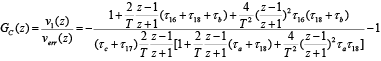where

τ16 = R3C2,

τ17 = R2C3,

τ18 = R1C1,

τa = R3C3,

τb = R2C1, and

τc = R2C2.

After defining the following intermediate variables,

a3 = 1 + 2/T(τ16 + τ18 + τb) + 4/T²τ16(τ18 + τb),

a2 = 3 + 2/T(τ16 + τ18 + τb) - 4/T²τ16(τ18 + τb),

a1 = 3 - 2/T(τ16 + τ18 + τb) - 4/T²τ16(τ18 + τb),

a0 = 1 - 2/T(τ16 + τ18 + τb) + 4/T²τ16(τ18 + τb),

b3 = 2/T(τc + τ17) + 4/T²(τc + τ17)(τa + τ18) + 8/T³(τc + τ17)τaτ18,

b2 = 2/T(τc + τ17) - 4/T²(τc + τ17)(τa + τ18) - 24/T³(τc + τ17)τaτ18,

b1 = -2/T(τc + τ17) - 4/T²(τc + τ17)(τa + τ18) + 24/T³(τc + τ17)τaτ18, and

b0 = -2/T(τc + τ17) + 4/T²(τc + τ17)(τa + τ18) - 8/T³(τc + τ17)τaτ18,

we can simplify Equation 23 to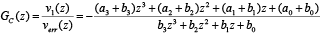After taking the inverse z-transform of Equation 24, we have Equation 9. The associated digital filter coefficients can be determined using the Excel calculator presented in Appendix B.

Similar to the process above, we can obtain the expression for GF(z). Plugging Equation 8 into Equation 4, we have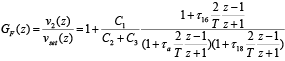After defining the following intermediate variables,

c2 = C1/(C2 + C3)(1 + (2/T)τ16),

c1 = 2C1/(C2 + C3),

c0 = C1/(C2 + C3)(1 - (2/T)τ16),

d2 = 1 + (2/T)(τa + τ18) + 4/T²τaτ18,

d1 = 2 - 8/T²τaτ18, and

d0 = 1 - 2/T(τa + τ18) + 4/T²τaτ18,

we can simplify Equation 25 to:After taking the inverse z-transform of Equation 26, we have Equation 10. The associated digital filter coefficients can be determined using the calculator presented in Appendix B.

### Appendix B: Calculating Digital Filter Coefficients

We have provided a calculator that can automatically calculate the digital filter coefficients in Equations 9, 10, and 15.

To determine the coefficients in Equations 9 and 10, one only needs to specify the values of R1, R2, R3, C1, C2, C3 (component functions shown in Figure 3), and T (the thermal-loop sampling period) near the top of the calculator. The coefficients (A's, B's, C's, and D's) will be generated near the bottom of the file, displayed in purple or yellow cells.

Similarly, one only needs to specify Kp, KI, and Tc in cells D88 to D90 to calculate the coefficients in Equation 15. This information will be provided in cells D92 to D94.

### Appendix C: Lab Results

We ran the example code on a slightly modified DS4830 EV board and captured the following transient responses shown in Figure 12. The yellow curve is the set-point voltage and the red curve is the thermistor voltage.

The experiment setup and procedures are as follows:

1. The DS4830 EV board with the following minor modifications:

• The change of R48, the TEC current sensing resistor, to 0.1Ω, 1%.
• The removal of R_TECC.
• The removal of R45, the on-board thermistor.
2. A transmitter optical subassembly (TOSA) mounted on a heat sink. The TOSA has a built-in TEC and thermistor.
3. DC power supplies:
• 3.3V for the EV board
• Variable DC supply for the set-point voltage
4. Connections:

• Connect TEC (+) of the TOSA to the R_TECC pad next to jumper LOADA on the EV board.
• Connect TEC (-) of the TOSA to the R_TECC pad next to jumper LOADB on the EV board.
• Connect the thermistor signal of the TOSA to test point TECC_TEMP on the EV board.
• Connect TOSA ground to EV board ground.
• Connect the set-point voltage to ADC_S14 (center pin of J7) on the EV board.
5. Power-on sequence:

• Power on the DS4830 EV kit.
• Turn on the set-point voltage with an initial value of 0.75V.
6. Testing the TEC control functionality:
• Decrease the set-point voltage to 0.40V and wait for the thermistor voltage to settle.
• Increase the set-point voltage back to 0.75V and wait for the thermistor voltage to settle.The TOSA used in this experiment has a typical operating temperature of 50°C, which corresponds to a 0.40V thermistor voltage. At room temperature, the thermistor voltage is about 0.75V. We captured the transient response of the thermistor voltage when the set-point voltage changes from 0.75V to 0.40V and then back to 0.75V. The rise time and the fall time are both very short and there is no overshoot. The data is shown in Table 6 below.

 Temperature Change Corresponding Set-Point Change Fall Time (90% to 10%) Fall Time (95% to 5%) Rise Time (90% to 10%) Rise Time (95% to 5%) 25°C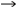50°C 0.75V0.40V 1.5s 1.8s N/A N/A 50°C25°C 0.40V0.75V N/A N/A 1.4s 2.1s

### Appendix D: Example Code

Sample DS4830 TEC control code (in C) is available for download. It should be noted that this code was customized for the lab setup described in Appendix C, which means that all the parameters, coefficients, and configurations are based on that particular setup. Users should NOT try running this code directly on their own TEC control board.